﻿ 大型舰船电气设备中电力变压器剩余寿命估计方法
 舰船科学技术2001, Vol. 44Issue (6): 165-169    DOI: 10.3404/j.issn.1672-7649.2022.06.035PDF

Residual life estimation method of power transformer in large ship electrical equipment
XIA Yun-peng
The 713 Research Institute of CSSC, Zhengzhou 450000, China
Abstract: In order to improve the accuracy of residual life estimation of power transformer in large ship electrical equipment, a residual life estimation method of power transformer in large ship electrical equipment is proposed. The remaining life estimation model of power transformer is constructed. The model is divided into three layers: the first layer estimates the health index of power transformer in large ship electrical equipment by using the basic information of power transformer. The second layer estimation model uses the test information of power transformer to obtain the aging state of power transformer in large ship electrical equipment; The initial health index of voltage transformer is determined by the first two-layer model. The third layer estimation model uses the operation information and fault information of power transformer to optimize the results of the first two layers estimation model to obtain the final health index of power transformer. According to the corresponding relationship between health index and residual life, the residual life estimation results of power transformer in large ship electrical equipment are obtained. The experimental results show that this method can accurately estimate the health index of voltage transformer and improve the estimation of residual life of power transformer.
Key words: power transformer     residual life     test information     operation information     fault information     health index
0 引　言

1 电力变压器剩余寿命估计方法 1.1 电力变压器剩余寿命估计模型构建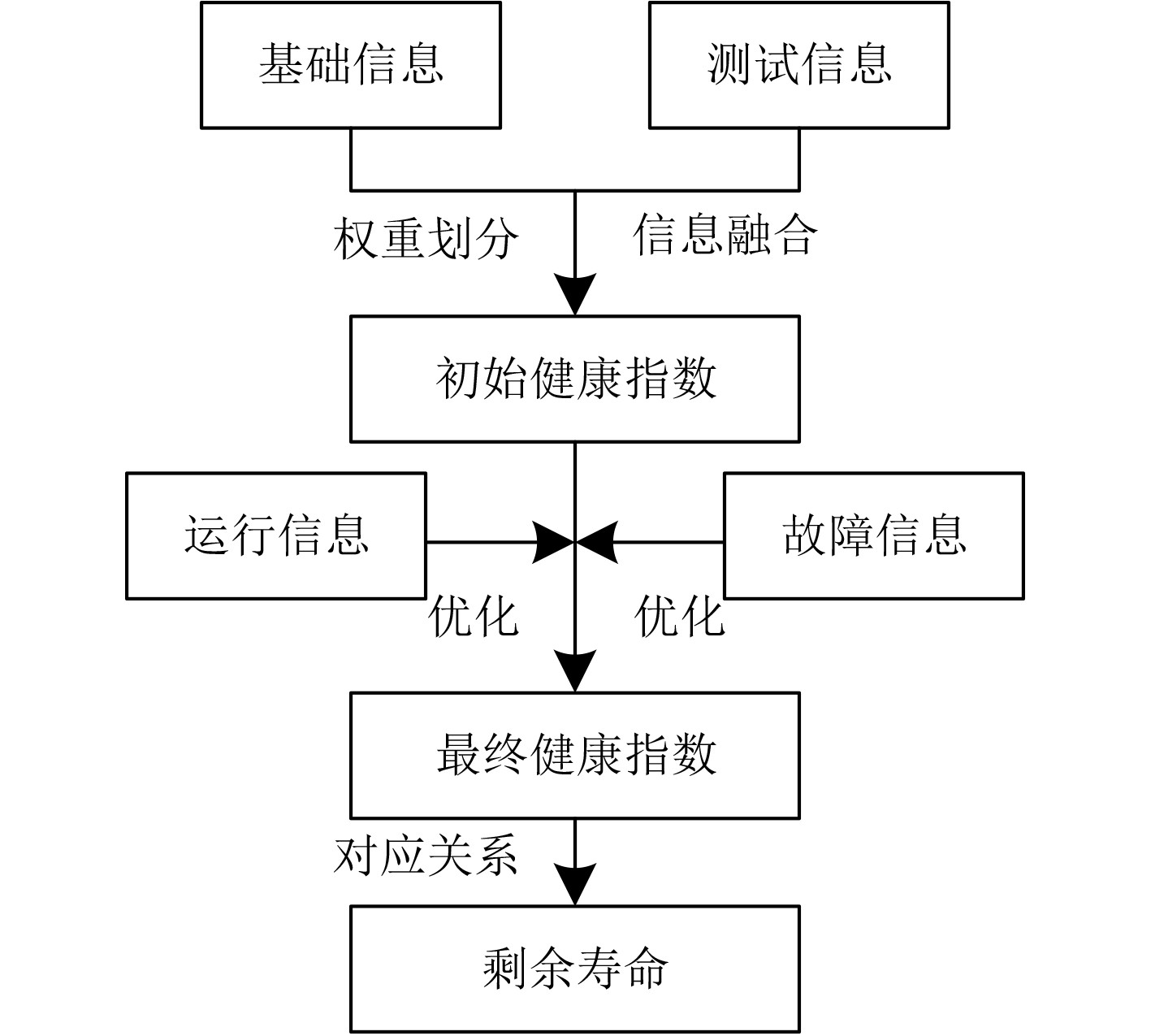图 1 剩余寿命估计模型 Fig. 1 Residual life estimation model

1.2 大型舰船电气设备中电力变压器健康指数 1.2.1 第一层估计模型

 $H{\text{ = }}{H_0} \cdot {e^{G\left( {{T_2} - {T_1}} \right)}} 。$ (1)

${T_d} = \left( {{T_t} - {T_1}} \right)$ 表示大型舰船电气设备中电力变压器预期寿命，其值由厂家处得到，由此能够获取 $G$ 的估计结果为：

 $G = \frac{{\ln \left( {{H_t} - {H_0}} \right)}}{{{T_d}}}。$ (2)

 $G = \frac{{\ln \left( {6.6 - 0.6} \right)}}{{{T_d}}} 。$ (3)

 ${H_j} = {H_0} \cdot {e^{G\left( {{T_2} - {T_1}} \right)}} = \frac{{{e^{G\left( {{T_2} - {T_1}} \right)}}}}{2} 。$ (4)
1.2.2 第二层估计模型

${H_{z1}}$ 表示油色谱测试所得数据，获取 ${H_{z1}}$ 的详细过程为：依照氢气、甲烷、乙烷、乙炔乙烯五类气体检测结果，分别确定气体的含量等级，以V表示。分别以各类气体检测所得气体含量等级与其气体检测对应的权重相乘，能够确定各类气体组分的分值，基于此得到油色谱测试结果为：

 $\left\{ \begin{gathered} {H_{z1}} = {S_{{{\rm{H}}_2}}} + {S_{{{\rm{C}}_2}{{\rm{H}}_6}}} + {S_{{\rm{C}}{{\rm{H}}_4}}} + {S_{{{\rm{C}}_2}{{\rm{H}}_4}}} + {S_{{{\rm{C}}_2}{{\rm{H}}_2}}} ，\hfill \\ {S_i} = {V_i}{W_i} 。\hfill \\ \end{gathered} \right.$ (5)

${H_{z2}}$ 表示糠醛测试所得数据，通过糠醛测试能够反映大型舰船电气设备中电力变压器绝缘的老化情况。通常情况下大型舰船电气设备中电力变压器内新的绝缘材料聚合度为1000，在其聚合度降至1/4的条件下需重点关注。纸聚合度 $DP$ 与糠醛含量间的相关性如下式：

 $DP = - 120 \cdot \ln {F_a} + 469。$ (6)

 ${H_{z2}} = 2.33{\left( {{F_a}} \right)^{0.68}} 。$ (7)

${H_{z3}}$ 表示油质测试所得数据，获取 ${H_{z3}}$ 的详细过程为：依照击穿水压、介质损耗与酸度的检测结果，分别确定不同检测项目的检测等级 $U$ ，并将各 $U$ 同其对应的权重相乘，由此得到不同气体组分分值，基于此得到油质测试结果为：

 $\left\{ \begin{gathered} {H_{z3}} = {S_a} + {S_b} + {S_c} ，\hfill \\ {S_i} = {U_i}{W_i} 。\hfill \\ \end{gathered} \right.$ (8)

${H_j}$ 为上限的条件下：

 ${H_c} = {H_j} \cdot {f_c} ；$ (9)

$H{I_j}$ 为非上限的条件下：

 ${H_c} = \max \left[ {\left( {{H_{z1}} \cdot {f_c}} \right) \cdot \left( {{H_{z2}} \cdot {f_c}} \right) \cdot \left( {{H_{z3}} \cdot {f_c}} \right)} \right] 。$ (10)

1.2.3 第三层估计模型

1）在大型舰船电气设备中电力变压器的投运时间不小于0年且不大于5年的条件下，投运时间优化因子 ${A_{11}}$ 值为1；在大型舰船电气设备中电力变压器的投运时间分别在大于5年且不大于10年、大于10年且不大于20年、大于20年且不大于30年和大于30年的条件下，投运时间优化因子 ${A_{11}}$ 值分别为1.01，1.02，1.05和1.09；

2）在铁芯接地电流值分别为0 A、大于0 A且不大于0.1 A、大于0.1 A且不大于0.3 A和大于0.3 A的条件下，铁芯接地电流优化因子 ${A_{12}}$ 值分别为1，1.05，1.1和1.2。

1）针对大型舰船电气设备中电力变压器本体、分接开关、冷却系统3个组件内外观等级最高的实施优化。大型舰船电气设备中电力变压器外观等级优化因子 ${A_{21}} = 0.9 + 0.1L\left( {L = 1,2, \cdots ,5} \right)$ ，其中，等级 $L$ 为1时表示大型舰船电气设备中电力变压器外观状态为最优状态。

2）大型舰船电气设备中电力变压器套管可靠等级 $R$ 可划分为5级，在 $R$ 取值分别为1～5时，可靠性优化值 ${A_R}$ 分别为0.9，1.0，1.1，1.2和1.4。以某型号三相电力变压器为例，其高、中、低侧套管的可靠性等级有所差异，如果 $\mathrm{max}\left({A}_{R高}，{A}_{R中}，{A}_{R低}\right)$ >1，则套管可靠等级优化因子 ${A}_{22}\text{=}{A}_{R高}+{A}_{R中}+{A}_{R低}$ ；如果 $\mathrm{max}\left({A}_{R高}，\right.$ $\left.{A}_{R中}，{A}_{R低}\right)$ ≤1，则套管可靠等级优化因子 ${A}_{22}\text{=} \mathrm{min}$ $\left({A}_{R高},{A}_{R中},{A}_{R低}\right)$

3）在大型舰船电气设备中电力变压器冷却系统采用油浸自冷与风冷方式时，冷却方式优化因子值为1；在大型舰船电气设备中电力变压器冷却系统，分别采用强迫油循环冷却方式和强迫导向油循环冷却方式时，冷却方式优化因子值 ${A_{23}}$ 分别为0.96和0.95。

4）在大型舰船电气设备中电力变压器近3年故障次数分别为0，1，2～4，5～10和高于10次的条件下，近3年故障次数优化因子 ${A_{24}}$ 分别取值0.96，1.00，1.04，1.20和1.40。

5）在大型舰船电气设备中电力变压器形成过近区短路时，近区短路优化因子 ${A_{25}}$ 取值1.04；相反 ${A_{25}}$ 取值1。

6）如果大型舰船电气设备中电力变压器产生局部放电问题，那么局部放电优化因子 ${A_{26}}$ 取值1.2；相反 ${A_{26}}$ 取值1。

 ${A_c} = \prod\limits_{m = 1}^2 {{A_{1m}}} \cdot \prod\limits_{n = 1}^6 {{A_{1n}}} ，$ (11)

 $H = {H_c}\frac{1}{{\left( {1 - {A_{1m}}} \right)}}{A_{1n}} 。$ (12)
1.3 基于健康指数的剩余寿命估计

 ${E_o} = \frac{{\dfrac{{\ln 7}}{I}}}{G} 。$ (13)
2 实验分析

2.1 健康指数估计结果表 1 研究对象历年健康指数 Tab.1 Health index of subjects over the years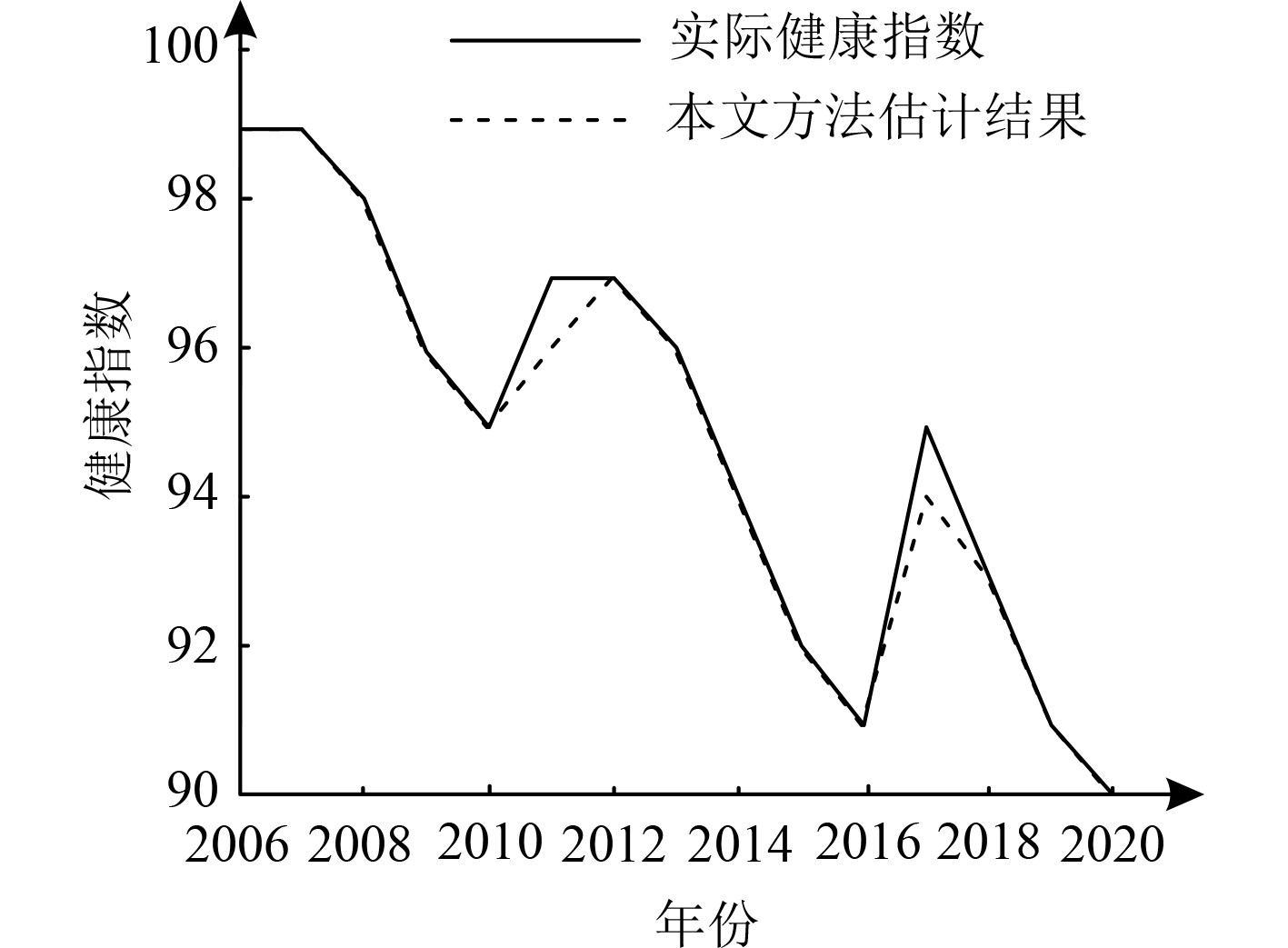图 2 健康指数估计精度 Fig. 2 Estimation accuracy of health index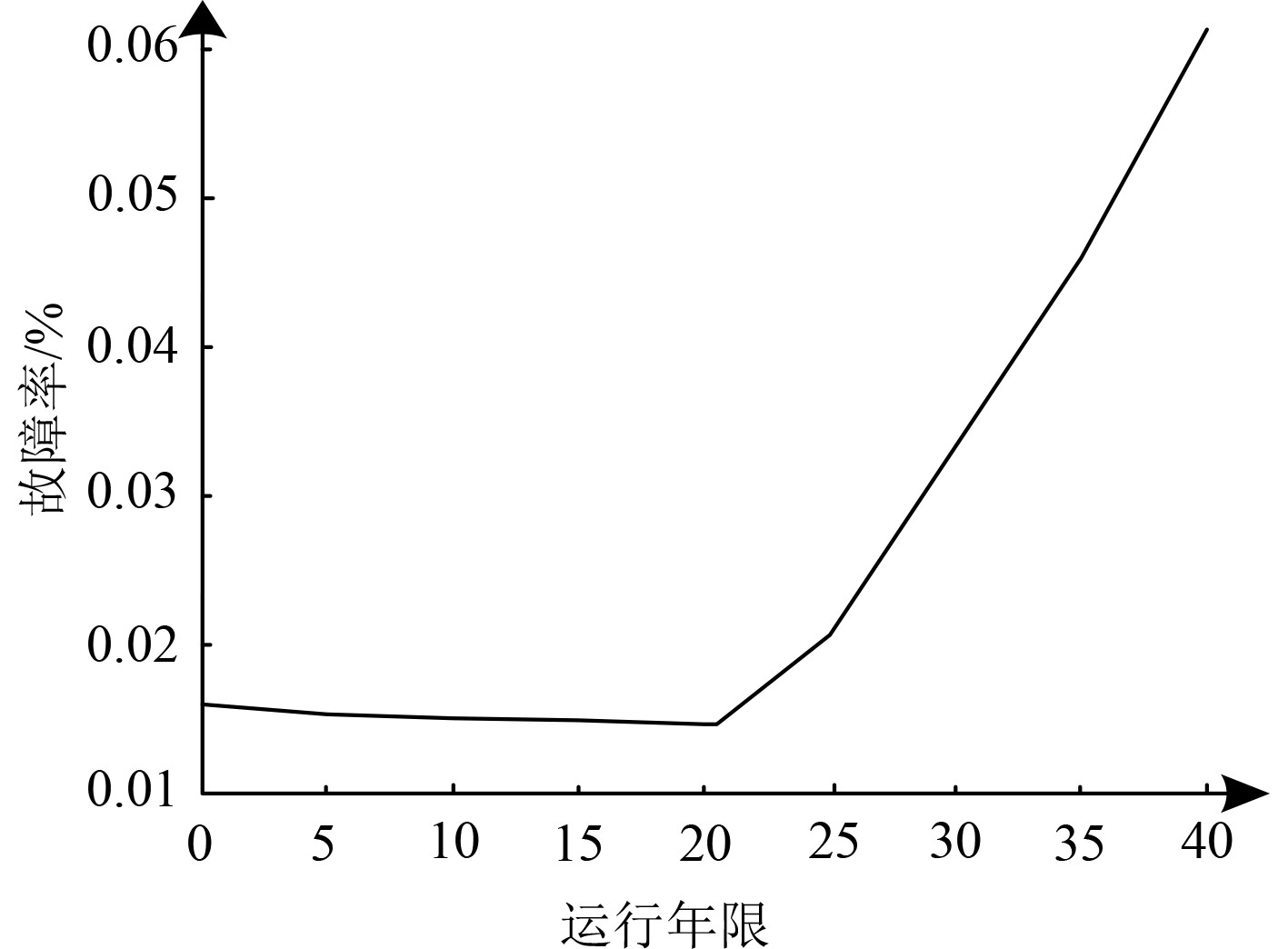图 3 故障率拟合曲线 Fig. 3 Fitting curve of failure rate

2.2 剩余寿命估计结果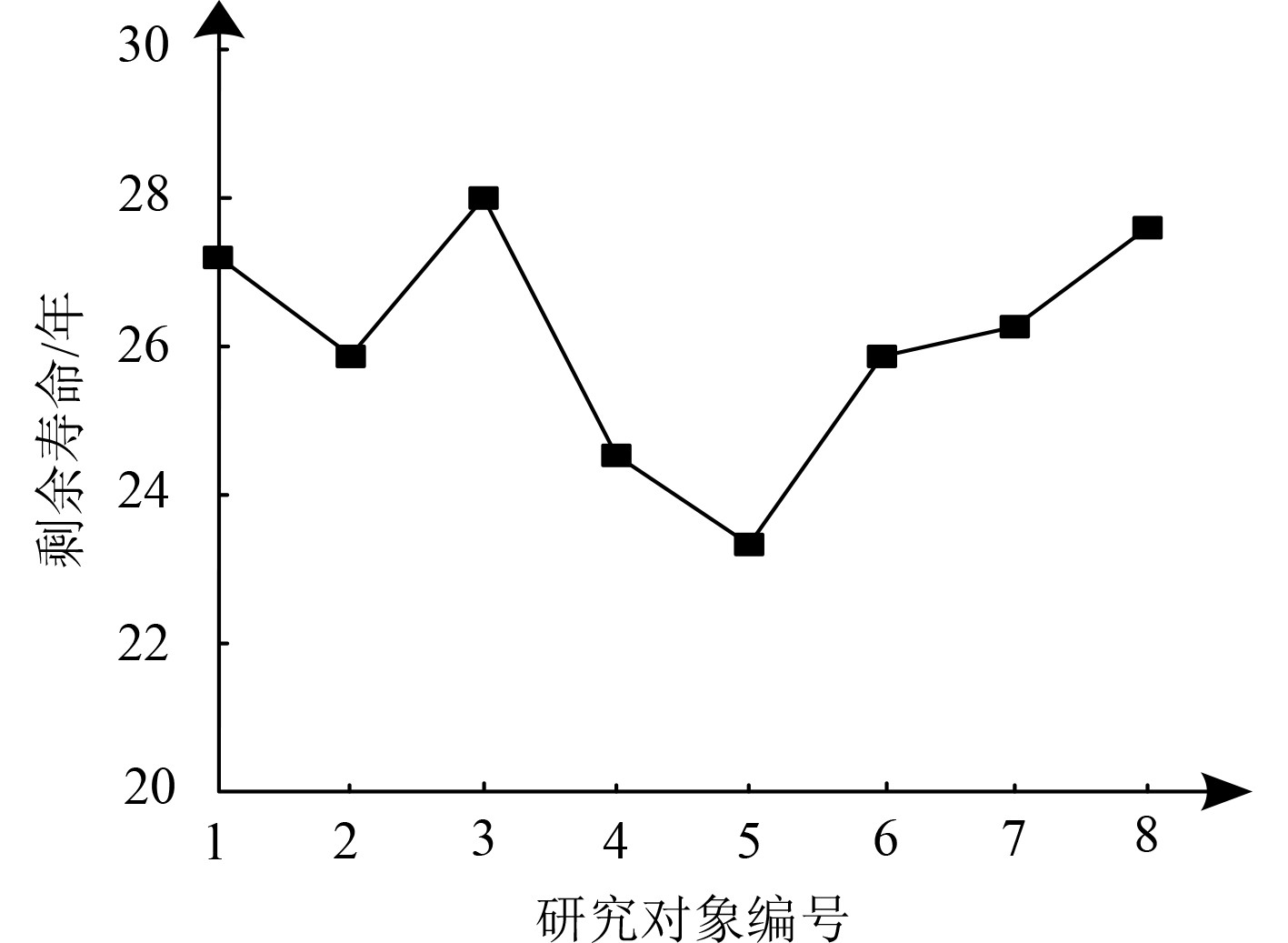图 4 各研究对象剩余寿命估计结果 Fig. 4 Residual life estimation results of each research object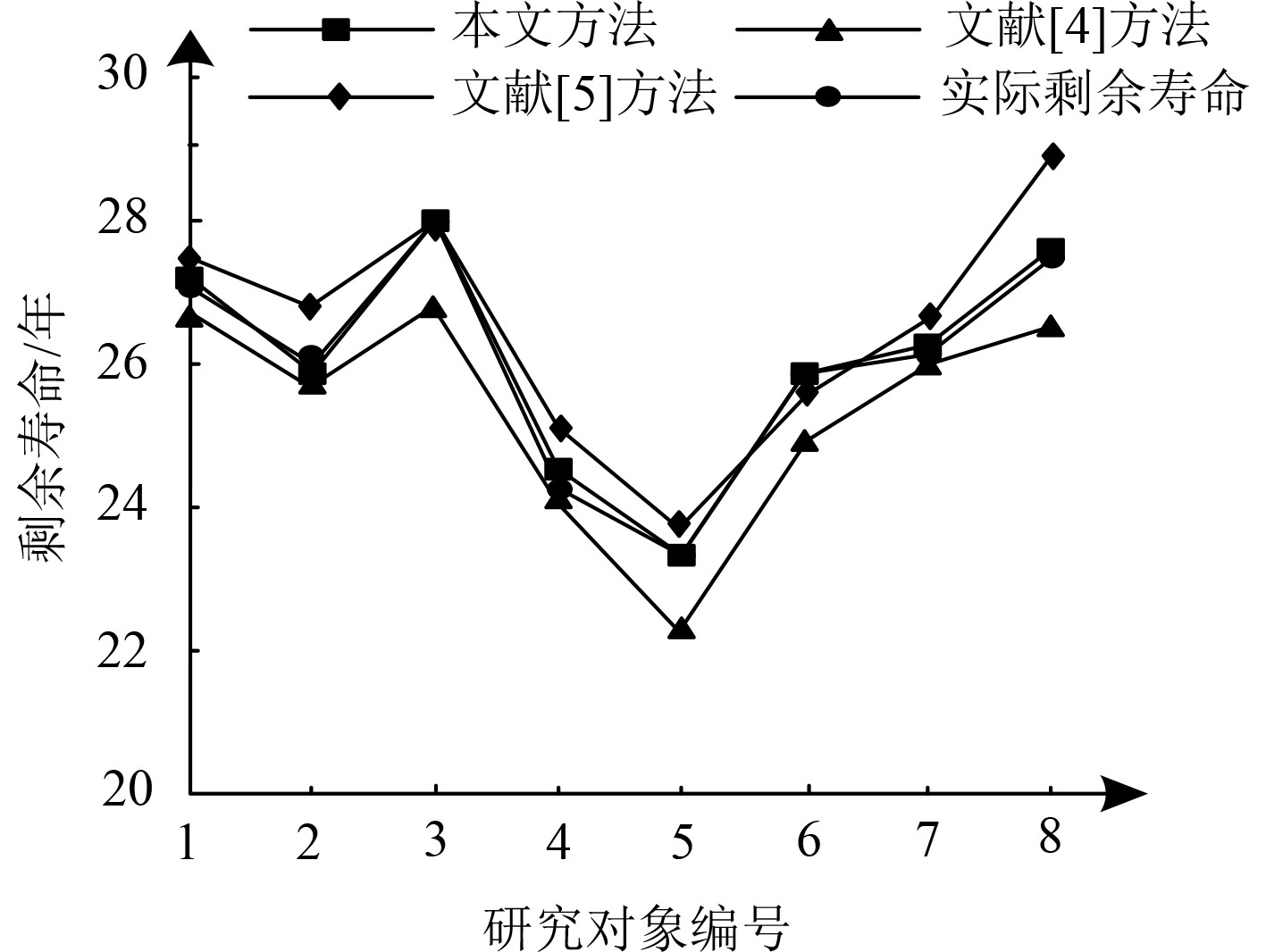图 5 研究对象剩余寿命对比结果 Fig. 5 Comparison results of remaining life of research objects

3 结　语

  刘云鹏, 许自强, 李刚, 等. 人工智能驱动的数据分析技术在电力变压器状态检修中的应用综述[J]. 高电压技术, 2019, 45(2): 337-348.  黄翔, 王凌云, 王泉, 等. 基于直觉模糊VIKOR法的电力变压器部件风险优先级评估[J]. 高压电器, 2020, 56(12): 177-183.  王威望, 刘莹, 何杰峰, 等. 高压大容量电力电子变压器中高频变压器研究现状和发展趋势[J]. 高电压技术, 2020, 46(10): 3362-3373.  陈长征, 刘洋. 基于隐马尔科夫模型的变压器剩余寿命分析[J]. 沈阳工业大学学报, 2020, 42(04): 412-416.  王文宾, 白文广, 石磊磊, 等. 电力变压器全寿命周期经济-物理综合寿命评估方法[J]. 电力系统保护与控制, 2019, 47(4): 91-98.  陈磊, 赵日升, 矫镕达, 等. 基于ANFIS和SOM的抽水蓄能电站主变压器健康状态监测[J]. 水电能源科学, 2020, 38(3): 177-180.  王利婷. 绝缘电阻的直接测量法及其在电气自动化工程中的应用——评《电力变压器智能故障诊断与绝缘测试技术》[J]. 绝缘材料, 2020, 53(11): 120.  张若愚, 齐波, 张鹏, 等. 面向电力变压器状态评价的油中溶解气体监测数据补全方法[J]. 电力自动化设备, 2019, 39(11): 181-187.  卞建鹏, 杨苏, 高世闯, 等. 基于全寿命周期成本的电力变压器检修决策[J]. 电力系统及其自动化学报, 2019, 31(5): 77-83.  谢志成, 钱海, 林湘宁, 等. 直流偏磁下变压器运行状态量化评估方法[J]. 电力自动化设备, 2019, 39(2): 216-223.  禹洪波, 袁婉玲, 汪敏, 等. 基于非对称贴近度证据云物元模型的电力变压器综合状态评估方法[J]. 电网技术, 2021, 45(9): 3706-3713.  王泉, 姚俊伟, 智李, 等. 基于矩估计法和模糊—证据融合的电力变压器套管状态评估[J]. 电力科学与技术学报, 2020, 35(1): 130-136.  鄢仁武, 罗家满, 徐育福. 基于盲数理论的电力变压器全寿命周期成本分析[J]. 电力系统及其自动化学报, 2019, 31(6): 15-20.  邓军, 孟杰, 潘志城, 等. 基于振动检测技术的电力变压器故障概率预测模型[J]. 变压器, 2020, 57(11): 37-40+68.